﻿ Launch of a Space Vehicle | Aerospace Engineering

## Launch of a Space Vehicle

### Orbit Meccanics:

The launch of a satellite or space vehicle consists of a period of powered flight during which the vehicle is lifted above the Earth’s atmosphere and accelerated to orbital velocity by a rocket, or launch v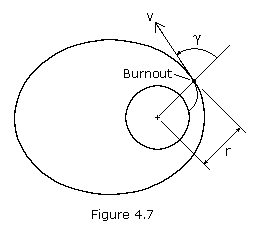ehicle. Powered flight concludes at burnout of the rocket’s last stage at which time the vehicle begins its free flight. During free flight the space vehicle is assumed to be subjected only to the gravitational pull of the Earth. If the vehicle moves far from the Earth, its trajectory may be affected by the gravitational influence of the sun, moon, or another planet.

A space vehicle’s orbit may be determined from the position and the velocity of the vehicle at the beginning of its free flight. A vehicle’s position and velocity can be described by the variables r, v, and, where r is the vehicle’s distance from the center of the Earth, v is its velocity, andis the angle between the position and the velocity vectors, called the zenith angle (see Figure 4.7). If we let  r1, v1, and1 be the initial (launch) values of  r, v, and, then we may consider these as given quantities. If we let point P2represent the perigee, then equation (4.13) becomes: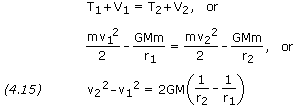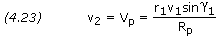Substituting equation (4.23) into (4.15), we can obtain an equation for the perigee radius Rp.Multiplying through by -Rp2/(r12v12) and rearranging, we get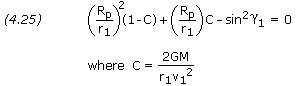Note that this is a simple quadratic equation in the ratio (Rp/r1) and that 2GM /(r1 × v12) is a nondimensional parameter of the orbit.

Solving for (Rp/r1) gives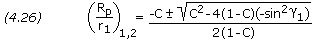Like any quadratic, the above equation yields two answers. The smaller of the two answers corresponds to Rp, the periapsis radius. The other root corresponds to the apoapsis radius, Ra.

Please note that in practice spacecraft launches are usually terminated at either perigee or apogee, i.e.= 90. This condition results in the minimum use of propellant.

```PROBLEM 1

A satellite is launched into Earth orbit where its launch vehicle burns out
at an altitude of 250 km.  At burnout the satellite's velocity is 7,900 m/s
with the zenith angle equal to 89 degrees.  Calculate the satellite's
altitude at perigee and apogee.

SOLUTION,

Given:  r1 = (6,378.14 + 250) × 1,000 = 6,628,140 m
v1 = 7,900 m/s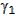= 89o

Equation (4.26),

(Rp / r1)1,2 = ( -C ± SQRT[ C2 - 4 × (1 - C) × -sin2]) / (2 ×
(1 - C))

where  C = 2 × GM / (r1 × v12)
C = 2 × 3.986005×1014 / (6,628,140 × 7,9002)
C = 1.927179

(Rp / r1)1,2 = ( -1.927179 ± SQRT[ 1.9271792 - 4 × -0.927179 × -
sin2(89) ]) / (2 × -0.927179)
(Rp / r1)1,2 = 0.996019 and 1.082521

Perigee Radius, Rp = Rp1 = r1 × (Rp / r1)1

Rp = 6,628,140 × 0.996019
Rp = 6,601,750 m

Altitude @ perigee = 6,601,750 / 1,000 - 6,378.14 = 223.6 km

Apogee Radius, Ra = Rp2 = r1 × (Rp / r1)2

Ra = 6,628,140 × 1.082521
Ra = 7,175,100 m

Altitude @ agogee = 7,175,100 / 1,000 - 6,378.14 = 797.0 km```

Equation (4.26) gives the values of Rp and Ra from which the eccentricity of the orbit can be calculated, however, it may be simpler to calculate the eccentricity e directly from the equation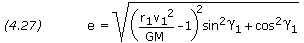```PROBLEM 2

Calculate the eccentricity of the orbit for the satellite in problem 1.

SOLUTION,

Given:  r1 = 6,628,140 m
v1 = 7,900 m/s= 89o

Equation (4.27),

e = SQRT[ (r1 × v12 / GM - 1)2 × sin2+ cos2]
e = SQRT[ (6,628,140 × 7,9002 / 3.986005×1014 - 1)2 × sin2(89) +
cos2(89) ]
e = 0.0416170```

To pin down a satellite’s orbit in space, we need to know the angle, the true anomaly, from the periapsis point to the launch point. This angle is given by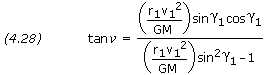```PROBLEM 3

Calculate the anglefrom perigee point to launch point for the
satellite in problem 1.

SOLUTION,

Given:  r1 = 6,628,140 m
v1 = 7,900 m/s= 89o

Equation (4.28),

tan= (r1 × v12 / GM) × sin× cos/
[(r1 × v12 / GM) × sin2- 1]
tan= (6,628,140 × 7,9002 / 3.986005×1014) × sin(89) × cos(89)
/ [(6,628,140 × 7,9002 / 3.986005×1014) × sin2(89) - 1]
tan= 0.48329= arctan(0.48329)= 25.794o```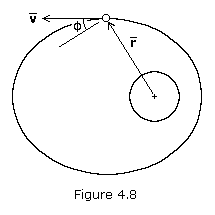In most calculations, the complement of the zenith angle is used, denoted by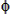. This angle is called the flight-path angle, and is positive when the velocity vector is directed away from the primary as shown in Figure 4.8. When flight-path angle is used, equations (4.26) through (4.28) are rewritten as follows: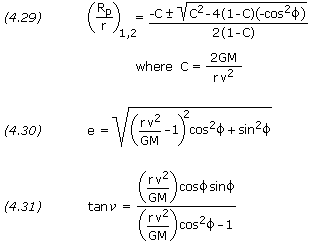The semi-major axis is, of course, equal to (Rp+Ra)/2, though it may be easier to calculate it directly as follows: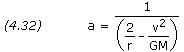```PROBLEM 4

Calculate the semi-major axis of the orbit for the satellite in problem 1.

SOLUTION,

Given:  r1 = 6,628,140 m
v1 = 7,900 m/s

Equation (4.32),

a = 1 / ( 2 / r1 - v12 / GM )
a = 1 / ( 2 / 6,628,140 - 7,9002 / 3.986005×1014) )
a = 6,888,430 m```

If e is solved for directly using equation (4.27) or (4.30), and a is solved for using equation (4.32), Rp and Ra can be solved for simply using equations (4.21) and (4.22).

Orbit Tilt, Rotation and Orientation

Above we determined the size and shape of the orbit, but to determine the orientation of the orbit in space, we must know the latitude and longitude and the heading of the space vehicle at burnout.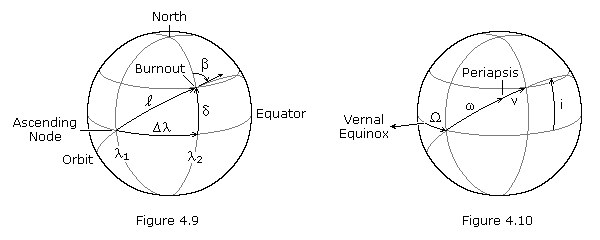Figure 4.9 above illustrates the location of a space vehicle at engine burnout, or orbit insertion.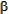is the azimuth heading measured in degrees clockwise from north,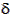is the geocentric latitude (or declination) of the burnout point,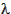is the angular distance between the ascending node and the burnout point measured in the equatorial plane, and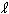is the angular distance between the ascending node and the burnout point measured in the orbital plane.1 and2 are the geographical longitudes of the ascending node and the burnout point at the instant of engine burnout. Figure 4.10 pictures the orbital elements, where i is the inclination,is the longitude at the ascending node,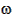is the argument of periapsis, andis the true anomaly.

If, and2 are given, the other values can be calculated from the following relationships: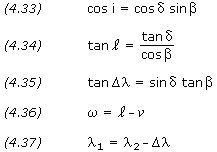In equation (4.36), the value ofis found using equation (4.28) or (4.31). Ifis positive, periapsis is west of the burnout point (as shown in Figure 4.10); ifis negative, periapsis is east of the burnout point.

The longitude of the ascending node,, is measured in celestial longitude, while1 is geographical longitude. The celestial longitude of the ascending node is equal to the local apparent sidereal time, in degrees, at longitude1 at the time of engine burnout. Sidereal time is defined as the hour angle of the vernal equinox at a specific locality and time; it has the same value as the right ascension of any celestial body that is crossing the local meridian at that same instant. At the moment when the vernal equinox crosses the local meridian, the local apparent sidereal time is 00:00. See this sidereal time calculator.

```PROBLEM 5

For the satellite in problem 4.8, burnout occurs 2000-10-20, 15:00 UT.  The
geocentric coordinates at burnout are 32o N latitude, 60o W longitude, and the
azimuth heading is 86o.  Calculate the orbit's inclination, argument of perigee,
and longitude of ascending node.

SOLUTION,

Given:= 86o= 32o2 = -60o

From problem 4.10,= 25.794o

Equation (4.33),

cos(i) = cos() × sin()
cos(i) = cos(32) × sin(86)
i = 32.223o

Equations (4.34) and (4.36),

tan() = tan() / cos()
tan() = tan(32) / cos(86)= 83.630o=-= 83.630 - 25.794= 57.836o

Equations (4.35) and (4.37),

tan() = sin() × tan()
tan() = sin(32) × tan(86)= 82.483o1 =2 -1 = -60 - 82.4831 = -142.483o= Sidereal time at -142.483 longitude, 2000-10-20, 15:00 UT= 7h 27' 34" = 111.892o```